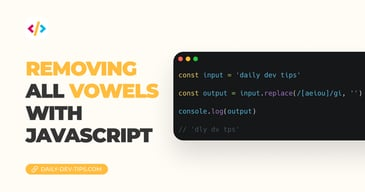Subscribe

# JavaScript basics loops

•••• 3 devs liked it

✍️

Looping code in JavaScript, understand the basic concepts of JavaScript

27 Aug, 2021 · 2 min read

In today's article on JavaScript basics, we'll be looking at different ways to create loops in JavaScript.

A loop is a way to iterate over code or execute code x times.

The different types of loops in JavaScript are:

• `for`
• `forEach`
• `for...in`
• `for...of`
• `while`
• `do...while`

I would say this is the godfather of loops. The basic for loop. Often you'll see this being used to loop over an array or execute code x times.

Let's first look at how we can create a loop that will execute five times.

``````for (let i = 0; i < 5; i++) {
// Execute 5 times
console.log(`This is loop number \${i}`);
}

// This is loop number 0
// This is loop number 1
// This is loop number 2
// This is loop number 3
// This is loop number 4``````

However, often we want to loop over an array of items. Let's say we have some foods and want to loop each view.

``````const foods = ['🍕', '🍗', '🍔', '🌮'];
for (let i = 0; i < foods.length; i++) {
console.log(foods[i]);
}

// 🍕
// 🍗
// 🍔
// 🌮``````

Ever since ES6 came out, we were introduced to the forEach method, making looping arrays way easier!

``````foods.forEach((item, index) => {
console.log(`\${index}: \${item}`);
});

// 0: 🍕
// 1: 🍗
// 2: 🍔
// 3: 🌮``````

Or as a one-liner:

``foods.forEach((item) => console.log(item));``

Another cool thing we can do is loop through the properties of an object!

Let's say we want to loop each property of this user object.

``````const user = {
firstName: 'Chris',
favoriteFood: '🍕',
};

for (let property in user) {
console.log(`\${property}: \${user[property]}`);
}

// firstName: Chris
// favoriteFood: 🍕``````

Then we also have the `for...of` loop, which can iterate over specific values instead of the properties.

``````const foods = ['🍕', '🍗', '🍔', '🌮'];
for (let value of foods) {
console.log(value);
}``````

The next big thing in loops is the `while` loop. This means code is executed while a condition is not met.

For instance, let's say we have a boolean value, and we should execute code until it's true.

``````let check = false;
while (!check) {
console.log('not correct');
check = true;
}``````

In this case, the code will execute once, be aware that this is a super-easy way to make an infinite loop that will crash your code!

With this, we can also evaluate a count, for instance, and only stop once the count is 5.

``````let amount = 0;
while (amount < 5) {
console.log(`amount \${amount}`);
amount++;
}

// amount 0
// amount 1
// amount 2
// amount 3
// amount 4``````

The `do...while` is very similar to the while loop, but the executing order differs.

Let's first look at how it works:

``````let test = true;
do {
console.log('testing');
test = false;
} while (test);

// testing``````

This will now execute once and evaluate that the test is not false. However, what happens when we start with the test being false?

``````let test = false;
do {
console.log('testing');
test = false;
} while (test);

// testing``````

Huh? This still logs testing. And yes it does The `do...while` loop executes the code and THEN evaluates the while statement. The `while` loop evaluates this code first before executing anything.

I hope you learned a thing or two about JavaScript loops!

I placed this code on a CodePen for you to check out and have a play around with.

See the Pen JavaScript basics loops by Chris Bongers (@rebelchris) on CodePen.

Tweet this tip
•••• 3 devs liked it

### Removing all vowels with JavaScript

3 Dec, 2022 · 2 min read### 10 games to learn JavaScript

2 Dec, 2022 · 3 min readJoin 2101 devs and subscribe to my newsletter

• 1000 articles written
• 2101 devs subscribed
• 529116 words written